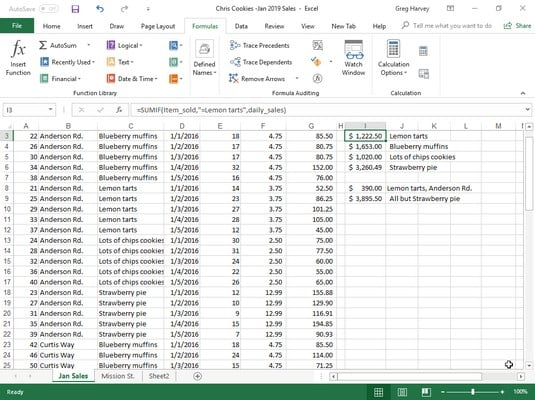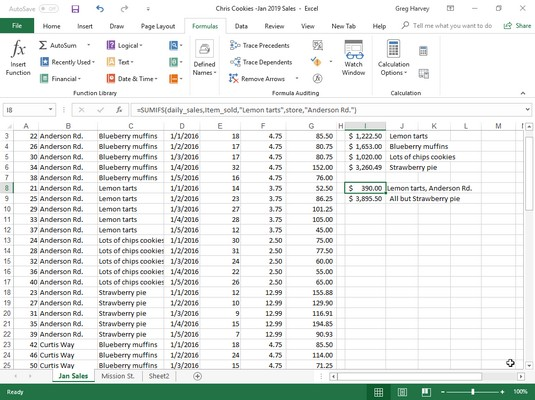##### Excel 2019 All-in-One For DummiesExcel functions can help you do great things. Excel’s SUM function is perfect when you want to get the totals for all the numbers in a particular range or set of ranges. But what about those times when you only want the total of certain items within a cell range? For those situations, you can use Excel’s SUMIF or SUMIFS function on the Math & Trig command button’s drop-down menu.

The SUMIF function enables you to tell Excel to add together the numbers in a particular range only when those numbers meet the criteria that you specify. The syntax of the SUMIF function is as follows:

`SUMIF(<em>range</em>,<em>criteria</em>,[<em>sum_range</em>])`
In the SUMIF function, the range argument specifies the range of cells that you want Excel to evaluate when doing the summing; the criteria argument specifies the criteria to be used in evaluating whether to include certain values in the range in the summing; and finally, the optional sum_range argument is the range of all the cells to be summed together. If you omit the sum_range argument, Excel sums only the cells specified in the range argument (and, of course, only if they meet the criteria specified in the criteria argument).

The SUMIFS (that’s ifs, plural) function works like SUMIF function except that it enables you to specify more than one criteria range that controls when a certain range of values are summed. Its syntax is a little bit different:

`SUMIFS(<em>sum_range,criteria_range,criteria,…)</em>`
For this function, the sum_range argument specifies all the possible values that can be summed, the criteria_range specifies the cells with all the entries that are to be evaluated by the if criteria, and the criteria argument contains the expression that is to be applied to the entries in the criteria_range to determine which of the values to total in the sum_range.

## Summing certain Excel cells with SUMIF

The image below illustrates how you can use the SUMIF function to total sales by the items sold. This shows a Sales data list sorted by the store location and then the item sold. In this Daily Sales data list, there are three locations: Mission Street, Anderson Rd., and Curtis Way, of which only sales made at the Anderson Rd. location are visible here.Using SUMIF to total sales by items sold.

To total the sales of Lemon tarts at all three locations in this data list, create the following SUMIF formula in cell I3:

`=SUMIF(item_sold,"=Lemon tarts",daily_sales)`
In this example, item_sold is the range name given to the cell range C3:C62, which contains the list of each item that has been sold in the first five days of January, 2016 (Lemon tarts, Blueberry muffins, Lots of chips cookies, or Strawberry pie), and daily_sales is the range name assigned to the cell range G3:G62, which contains the extended sales made at each store for each item.

The SUMIF formula in cell I3 then looks for each occurrence of “Lemon tarts” in the item_sold range (the criteria argument for the SUMIF function) in the Item column of the Cookie Sales list and then adds its extended sales price from the daily_sales range in the Daily Sales column to the total.

The formulas in cells I4, I5, and I6 contain SUMIF functions very similar to the one in cell I3, except that they substitute the name of the dessert goodie in question in place of the =Lemon tarts criteria argument.

## Summing on multiple criteria with SUMIFS

This image illustrates the use of the SUMIFS function to apply multiple criteria in the summing of the daily sales. Here, you want to know the total of the sales of one item (Lemon tarts) at one store location (Anderson Rd.).Using SUMIFS to total sales by location as well as the items sold.

In order to do this, the following formula in cell I8 was created using the SUMIFS function:

`=SUMIFS(daily_sales,item_sold,"Lemon tarts",store,"Anderson Rd.")`
In this formula, the sum_range argument (specified first and not last as in SUMIF) is still the daily_sales cell range (G3:G62). The first criteria_range argument is item_sold (C3:C62) where the criteria is “Lemon tarts,” and the second criteria_range argument is store (B3:B62) where the criteria is “Anderson Rd.” When Excel evaluates the formula in cell I8, it applies both criteria so that the program ends up totaling only those daily sales where the item is Lemon tarts and the store location is Anderson Rd.

The formula in cell I9 immediately below in the worksheet shown above also uses the SUMIFS function, but this time applies just a single criteria in performing the summation. This formula sums the daily sales for any bakery item that is not a Strawberry pie:

`=SUMIFS(daily_sales,item_sold,"<>Strawberry pie")`
Because the item Strawberry pie was prefaced with the not (<>) operator (which can be placed before or after the open double quotation mark), Excel sums the sale of every item except for Strawberry pie.